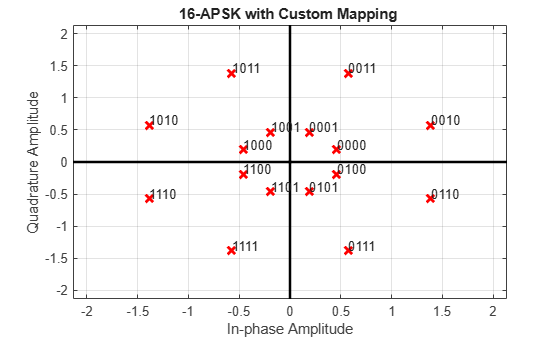## Amplitude and Phase Modulation Examples

These examples demonstrate amplitude and phase modulation techniques.

### Apply APSK Modulation Modifying Symbol Ordering

Plot APSK constellations for Gray-coded and custom-coded symbol mappings.

Define vectors for modulation order and PSK ring radii. Generate bit data for constellation points.

```M = [8 8]; modOrder = sum(M); radii = [0.5 1.5]; x = 0:modOrder-1;```

The `apskmod` function assumes the single channel binary input is left-MSB aligned and specified column-wise. Use the `int2bit` function to express the integer input symbols as a single column binary vector.

`xBit = int2bit(x,log2(modOrder));`

Apply APSK modulation to the data using the default phase offset. Since element values for `M` are equal and element values for phase offset are equal, the symbol mapping defaults to `'gray'`. Plot the constellation using binary input to highlight the Gray-coded nature of the constellation mapping.

`y = apskmod(xBit,M,radii,PlotConstellation=true,InputType='bit');`Create a custom-coded symbol mapping vector. This custom mapping happens to be another Gray-coded mapping.

`cmap = [0;1;9;8;12;13;5;4;2;3;11;10;14;15;7;6];`

Apply APSK modulation with a custom-coded symbol mapping. Plot the constellation using binary input to highlight that the custom mapping defines different Gray-coded symbol mapping.

```z = apskmod(xBit,M,radii, ... SymbolMapping=cmap, ... PlotConstellation=true, ... InputType='bit');```### Demodulate MIL-STD-188-110C Specific 64-QAM Signal

Demodulate a 64-QAM signal that was modulated as specified in MIL-STD-188-110C. Compute hard decision bit output and verify that the output matches the input.

Set the modulation order and generate random bit data.

```M = 64; numBitsPerSym = log2(M); x = randi([0 1],1000*numBitsPerSym,1);```

Modulate the data. Use name-value pairs to specify bit input data and to plot the constellation.

`txSig = mil188qammod(x,M,'InputType','bit','PlotConstellation',true);`Demodulate the received signal. Compare the demodulated data to the original data.

```z = mil188qamdemod(txSig,M,'OutputType','bit'); isequal(z,x)```
```ans = logical 1 ```

### Plot Noisy DVB-S APSK Constellation using Simulink

Apply DVB-S APSK modulation to random data symbols, pass the modulated signal through an AWGN channel, and then plot the signal constellation.The `slex_dvbs_apsk_mod` model is configured for the S2 standard suffix and modulation order 16. For you to get desired results, all blocks in the model must align their configuration to the same modulation order. To set the modulation order for the DVBS-APSK Modulator Baseband block, select a value from the dropdown list in the block mask. The set of modulation orders available varies depending on the DVB standard suffix parameter setting. The `InitFcn` callback defines the workspace variable, `M` = 16, and this variable is used to set the:

The Constellation Diagram block has the Reference constellation parameter set to Custom and the Custom value parameter set to align with the modulator block's modulation setting. To see the values, open Reference Constellation in the Plot tab on the Constellation Diagram window.

Run the model with the EbN0 of the AWGN channel block set to 20 dB, and then view the constellation diagram.Change the EbN0 of the AWGN channel block to 10 dB. Run the model and observe the increase in noise shown in the plotted constellation diagram.You can try running the model with a different modulation order. To adjust the modulation order, you must align the:

• Modulation order setting in the DVBS-APSK Modulator Baseband block

• Value of the workspace variable `M`

• Reference constellation in the Constellation Diagram block

If these parameters are not aligned with each other, the reference constellation and input signal do not produce the desired constellation.

### Demodulate Noisy 16-APSK Signal Using Simulink

Apply 16-APSK modulation to a signal of random data. Pass the modulated signal through an AWGN channel. Demodulate the noisy 16-APSK signal. Check the bit error rate (BER).

The `slex_16apsk_mod` model passes a 16-APSK modulated signal through an AWGN channel, demodulates the signal and then comuptes the error rate statistics. The example reports the bit error rate (BER) at two EbN0 settings.Run the model with the EbN0 of the AWGN channel block set to 6 dB. The results are saved to the base workspace variable `ErrorVec` in a 1-by-3 row vector. The first element contains the BER.

```With EbN0 set to 6 dB, BER: 0.164 ```

Change the EbN0 of the AWGN channel block to 10 dB. Run the model and observe the decrease in BER.

```With EbN0 set to 10 dB, BER: 0.012 ```